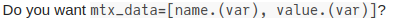# Julia exits when running several models (JuMP) in a row

Hi,
I’m running several instances on the same model in order to compare performance, like described below.
My problem is that I have 1000 instances and halfway Julia exit, probably due to memory, but I don’t really understand why. What am I missing?
Just to clarify, this is a simpler version, “df_results” have more columns but the end file is less than 1 Mb.
Only “vec_data” ends up a bit larger with around 10Mb, still not a lot.
Inside the function the model (MIPL) is made with JuMP and solved (Gurobi) returning a table with variable & value and a vector with data for the results (time, value, etc).
Thank you!

``````# Environment
const GUROBI_ENV = Gurobi.Env()
# Intances to be solved (DataFrame)
instances
# Conditions to be tested (Vector)
conditions
# DataFrame to store results
df_results = DataFrame(instance=0,
solve_time=0,
objective_value=0)
# vector to store resultins model variables
vec_data = []
# to loop the instances
for inst in instances
for cond in condition
# Running model
results, data = model_function(inst,cond)
# Pushin results and data
push!(vec_data,data)
push!(df_results,results)
# Saving
CSV.write("/home/result.csv",df_results)
f = open("/home/data.jl", "w")
print(f, vec_data)
close(f)
end
end

``````

It’s hard to say what’s going on without a reproducible example. Can you add more detail to your post? How are you creating the models? What is `model_function`? etc.

What is `data`? If it contains a model variable, then the variable also has a reference to the model, and so everything will be kept in memory.

Do you have a log from the REPL? Was any error thrown before exiting?

1 Like

I will edit the post to add some more info by what you commented, being honest I was unsure what would help. I was thinking it might be memory because I can run the 1000 instances in 2 batches.
Is there any specific information that might be important for the model? I can’t share the whole model so I have to cut some info.
About the error, I will run again to get a print but it is just a red line saying that Julia exited.
When I get all the info I will update the post.
Thanks

Is there any specific information that might be important for the model?

What is `data`? If it contains a model variable, then the variable also has a reference to the model, and so everything will be kept in memory.

That might be it.
The “data” I get from the function is:

``````var = all_variables(modelo)
mtx_data=[var,value.(var)]
# This is returned as "data"
df_data=DataFrame(mtx_data, ["var_name","var_value"])
``````

By what you said the “value.(var)” or “var” should still be referencing the model, right?
Would it work if I try and convert to numeric and string?

Do you want `mtx_data=[name.(var), value.(var)]`?

should still be referencing the model, right?

Yes. This is the problem. You’re keeping a reference to every variable and every model, so Julia cannot free any memory.Yes, that should solve it.
I will run one more just to be sure.
Thank you.

1 Like